21 de Feb de 2023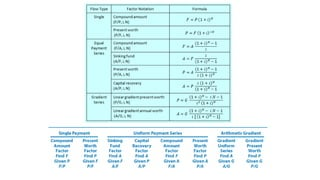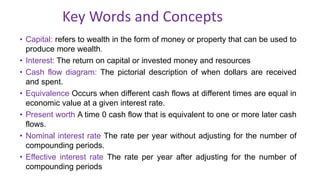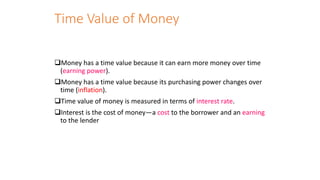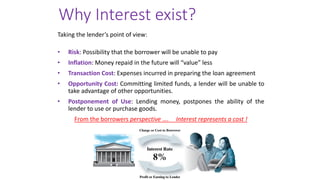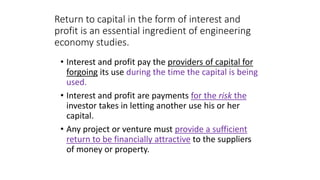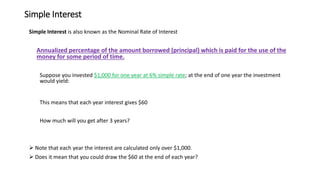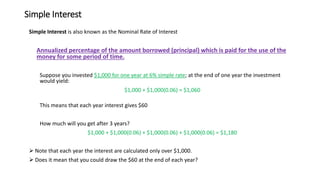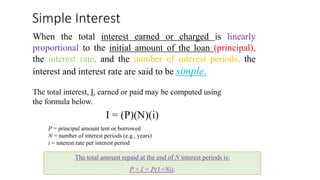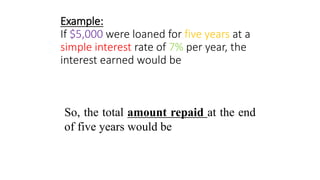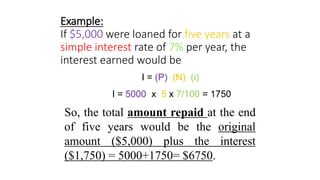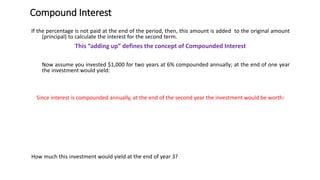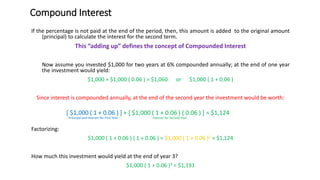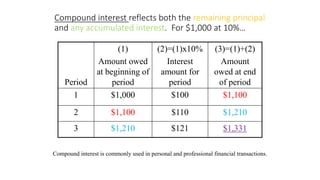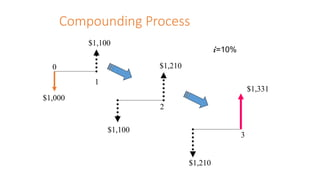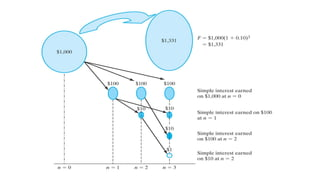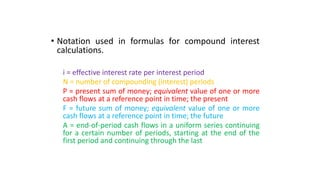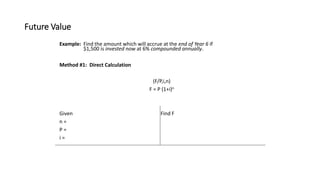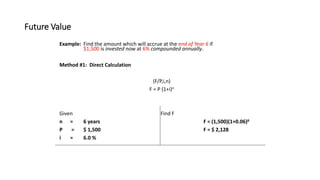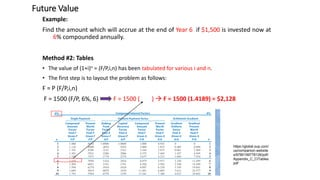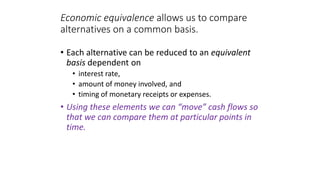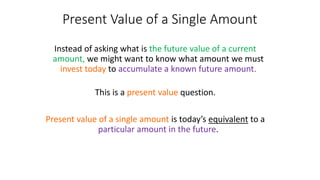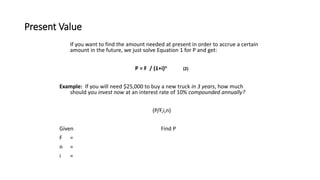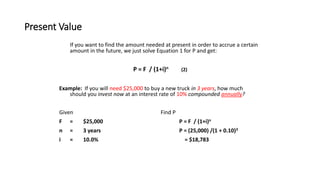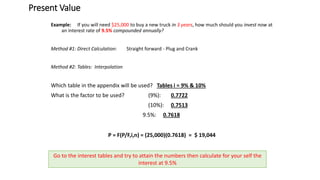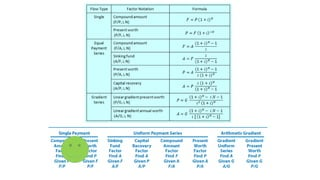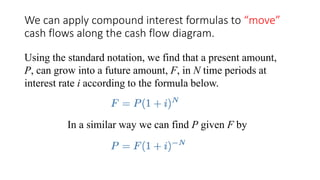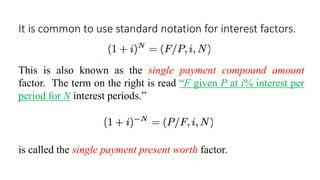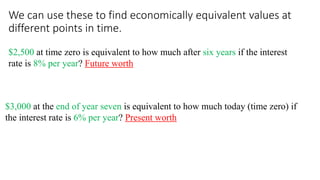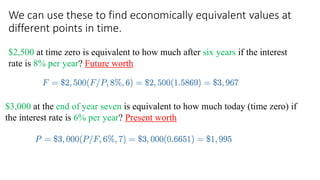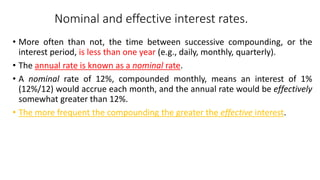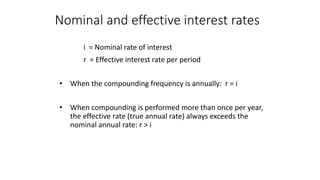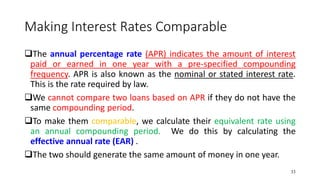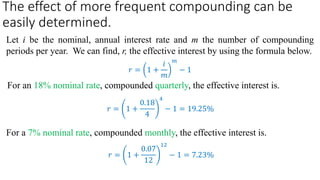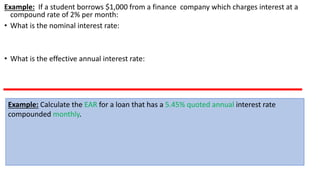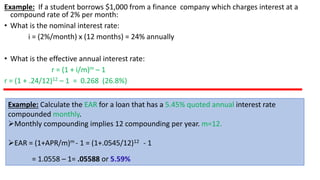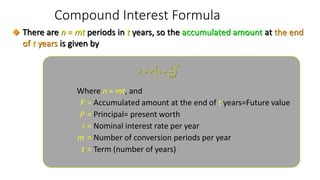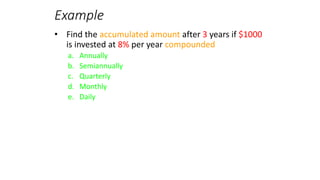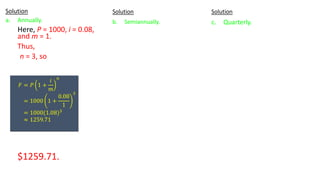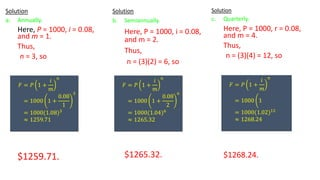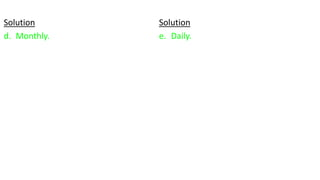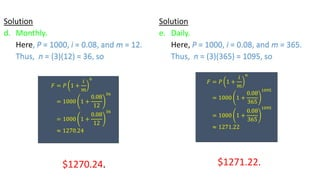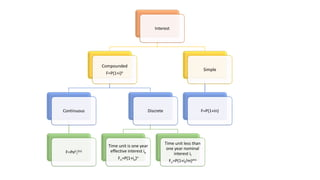1 de 43

• 1. Engineering Management, Economics and Risk Analysis Dr Jeb Gholinezhad
• 3. Key Words and Concepts • Capital: refers to wealth in the form of money or property that can be used to produce more wealth. • Interest: The return on capital or invested money and resources • Cash flow diagram: The pictorial description of when dollars are received and spent. • Equivalence Occurs when different cash flows at different times are equal in economic value at a given interest rate. • Present worth A time 0 cash flow that is equivalent to one or more later cash flows. • Nominal interest rate The rate per year without adjusting for the number of compounding periods. • Effective interest rate The rate per year after adjusting for the number of compounding periods
• 4. Time Value of Money ❑Money has a time value because it can earn more money over time (earning power). ❑Money has a time value because its purchasing power changes over time (inflation). ❑Time value of money is measured in terms of interest rate. ❑Interest is the cost of money—a cost to the borrower and an earning to the lender
• 5. Why Interest exist? Taking the lender’s point of view: • Risk: Possibility that the borrower will be unable to pay • Inflation: Money repaid in the future will “value” less • Transaction Cost: Expenses incurred in preparing the loan agreement • Opportunity Cost: Committing limited funds, a lender will be unable to take advantage of other opportunities. • Postponement of Use: Lending money, postpones the ability of the lender to use or purchase goods. From the borrowers perspective …. Interest represents a cost !
• 6. Return to capital in the form of interest and profit is an essential ingredient of engineering economy studies. • Interest and profit pay the providers of capital for forgoing its use during the time the capital is being used. • Interest and profit are payments for the risk the investor takes in letting another use his or her capital. • Any project or venture must provide a sufficient return to be financially attractive to the suppliers of money or property.
• 7. Simple Interest Simple Interest is also known as the Nominal Rate of Interest Annualized percentage of the amount borrowed (principal) which is paid for the use of the money for some period of time. Suppose you invested \$1,000 for one year at 6% simple rate; at the end of one year the investment would yield: This means that each year interest gives \$60 How much will you get after 3 years? ➢ Note that each year the interest are calculated only over \$1,000. ➢ Does it mean that you could draw the \$60 at the end of each year?
• 8. Simple Interest Simple Interest is also known as the Nominal Rate of Interest Annualized percentage of the amount borrowed (principal) which is paid for the use of the money for some period of time. Suppose you invested \$1,000 for one year at 6% simple rate; at the end of one year the investment would yield: \$1,000 + \$1,000(0.06) = \$1,060 This means that each year interest gives \$60 How much will you get after 3 years? \$1,000 + \$1,000(0.06) + \$1,000(0.06) + \$1,000(0.06) = \$1,180 ➢ Note that each year the interest are calculated only over \$1,000. ➢ Does it mean that you could draw the \$60 at the end of each year?
• 9. Simple Interest When the total interest earned or charged is linearly proportional to the initial amount of the loan (principal), the interest rate, and the number of interest periods, the interest and interest rate are said to be simple. The total interest, I, earned or paid may be computed using the formula below. I = (P)(N)(i) P = principal amount lent or borrowed N = number of interest periods (e.g., years) i = interest rate per interest period The total amount repaid at the end of N interest periods is: P + I = P(1+Ni).
• 10. Example: If \$5,000 were loaned for five years at a simple interest rate of 7% per year, the interest earned would be So, the total amount repaid at the end of five years would be
• 11. Example: If \$5,000 were loaned for five years at a simple interest rate of 7% per year, the interest earned would be So, the total amount repaid at the end of five years would be the original amount (\$5,000) plus the interest (\$1,750) = 5000+1750= \$6750. I = (P) (N) (i) I = 5000 x 5 x 7/100 = 1750
• 12. Compound Interest If the percentage is not paid at the end of the period, then, this amount is added to the original amount (principal) to calculate the interest for the second term. This “adding up” defines the concept of Compounded Interest Now assume you invested \$1,000 for two years at 6% compounded annually; at the end of one year the investment would yield: Since interest is compounded annually, at the end of the second year the investment would be worth: How much this investment would yield at the end of year 3?
• 13. Compound Interest If the percentage is not paid at the end of the period, then, this amount is added to the original amount (principal) to calculate the interest for the second term. This “adding up” defines the concept of Compounded Interest Now assume you invested \$1,000 for two years at 6% compounded annually; at the end of one year the investment would yield: \$1,000 + \$1,000 ( 0.06 ) = \$1,060 or \$1,000 ( 1 + 0.06 ) Since interest is compounded annually, at the end of the second year the investment would be worth: [ \$1,000 ( 1 + 0.06 ) ] + [ \$1,000 ( 1 + 0.06 ) ( 0.06 ) ] = \$1,124 Principal and Interest for First Year Interest for Second Year Factorizing: \$1,000 ( 1 + 0.06 ) ( 1 + 0.06 ) = \$1,000 ( 1 + 0.06 )2 = \$1,124 How much this investment would yield at the end of year 3? \$1,000 ( 1 + 0.06 )3 = \$1,191
• 14. Compound interest reflects both the remaining principal and any accumulated interest. For \$1,000 at 10%… Period (1) Amount owed at beginning of period (2)=(1)x10% Interest amount for period (3)=(1)+(2) Amount owed at end of period 1 \$1,000 \$100 \$1,100 2 \$1,100 \$110 \$1,210 3 \$1,210 \$121 \$1,331 Compound interest is commonly used in personal and professional financial transactions.
• 17. • Notation used in formulas for compound interest calculations. i = effective interest rate per interest period N = number of compounding (interest) periods P = present sum of money; equivalent value of one or more cash flows at a reference point in time; the present F = future sum of money; equivalent value of one or more cash flows at a reference point in time; the future A = end-of-period cash flows in a uniform series continuing for a certain number of periods, starting at the end of the first period and continuing through the last
• 18. Future Value Example: Find the amount which will accrue at the end of Year 6 if \$1,500 is invested now at 6% compounded annually. Method #1: Direct Calculation (F/P,i,n) F = P (1+i)n Given Find F n = P = i =
• 19. Future Value Example: Find the amount which will accrue at the end of Year 6 if \$1,500 is invested now at 6% compounded annually. Method #1: Direct Calculation (F/P,i,n) F = P (1+i)n Given Find F n = 6 years F = (1,500)(1+0.06)6 P = \$ 1,500 F = \$ 2,128 i = 6.0 %
• 20. Future Value Example: Find the amount which will accrue at the end of Year 6 if \$1,500 is invested now at 6% compounded annually. Method #2: Tables • The value of (1+i)n = (F/P,i,n) has been tabulated for various i and n. • The first step is to layout the problem as follows: F = P (F/P,i,n) F = 1500 (F/P, 6%, 6) F = 1500 ( ) → F = 1500 (1.4189) = \$2,128 https://global.oup.com/ us/companion.website s/9780199778126/pdf/ Appendix_C_CITables. pdf
• 21. Economic equivalence allows us to compare alternatives on a common basis. • Each alternative can be reduced to an equivalent basis dependent on • interest rate, • amount of money involved, and • timing of monetary receipts or expenses. • Using these elements we can “move” cash flows so that we can compare them at particular points in time.
• 22. Present Value of a Single Amount Instead of asking what is the future value of a current amount, we might want to know what amount we must invest today to accumulate a known future amount. This is a present value question. Present value of a single amount is today’s equivalent to a particular amount in the future.
• 23. Present Value If you want to find the amount needed at present in order to accrue a certain amount in the future, we just solve Equation 1 for P and get: P = F / (1+i)n (2) Example: If you will need \$25,000 to buy a new truck in 3 years, how much should you invest now at an interest rate of 10% compounded annually? (P/F,i,n) Given Find P F = n = i =
• 24. Present Value If you want to find the amount needed at present in order to accrue a certain amount in the future, we just solve Equation 1 for P and get: P = F / (1+i)n (2) Example: If you will need \$25,000 to buy a new truck in 3 years, how much should you invest now at an interest rate of 10% compounded annually? Given Find P F = \$25,000 P = F / (1+i)n n = 3 years P = (25,000) /(1 + 0.10)3 i = 10.0% = \$18,783
• 25. Present Value Example: If you will need \$25,000 to buy a new truck in 3 years, how much should you invest now at an interest rate of 9.5% compounded annually? Method #1: Direct Calculation: Straight forward - Plug and Crank Method #2: Tables: Interpolation Which table in the appendix will be used? Tables i = 9% & 10% What is the factor to be used? (9%): 0.7722 (10%): 0.7513 9.5%: 0.7618 P = F(P/F,i,n) = (25,000)(0.7618) = \$ 19,044 Go to the interest tables and try to attain the numbers then calculate for your self the interest at 9.5%
• 27. We can apply compound interest formulas to “move” cash flows along the cash flow diagram. Using the standard notation, we find that a present amount, P, can grow into a future amount, F, in N time periods at interest rate i according to the formula below. In a similar way we can find P given F by
• 28. It is common to use standard notation for interest factors. This is also known as the single payment compound amount factor. The term on the right is read “F given P at i% interest per period for N interest periods.” is called the single payment present worth factor.
• 29. We can use these to find economically equivalent values at different points in time. \$2,500 at time zero is equivalent to how much after six years if the interest rate is 8% per year? Future worth \$3,000 at the end of year seven is equivalent to how much today (time zero) if the interest rate is 6% per year? Present worth
• 30. We can use these to find economically equivalent values at different points in time. \$2,500 at time zero is equivalent to how much after six years if the interest rate is 8% per year? Future worth \$3,000 at the end of year seven is equivalent to how much today (time zero) if the interest rate is 6% per year? Present worth
• 31. Nominal and effective interest rates. • More often than not, the time between successive compounding, or the interest period, is less than one year (e.g., daily, monthly, quarterly). • The annual rate is known as a nominal rate. • A nominal rate of 12%, compounded monthly, means an interest of 1% (12%/12) would accrue each month, and the annual rate would be effectively somewhat greater than 12%. • The more frequent the compounding the greater the effective interest.
• 32. i = Nominal rate of interest r = Effective interest rate per period • When the compounding frequency is annually: r = i • When compounding is performed more than once per year, the effective rate (true annual rate) always exceeds the nominal annual rate: r > i Nominal and effective interest rates
• 33. Making Interest Rates Comparable ❑The annual percentage rate (APR) indicates the amount of interest paid or earned in one year with a pre-specified compounding frequency. APR is also known as the nominal or stated interest rate. This is the rate required by law. ❑We cannot compare two loans based on APR if they do not have the same compounding period. ❑To make them comparable, we calculate their equivalent rate using an annual compounding period. We do this by calculating the effective annual rate (EAR) . ❑The two should generate the same amount of money in one year. 33
• 34. The effect of more frequent compounding can be easily determined. Let i be the nominal, annual interest rate and m the number of compounding periods per year. We can find, r, the effective interest by using the formula below. For an 18% nominal rate, compounded quarterly, the effective interest is. For a 7% nominal rate, compounded monthly, the effective interest is. 𝑟 = 1 + 𝑖 𝑚 𝑚 − 1 𝑟 = 1 + 0.18 4 4 − 1 = 19.25% 𝑟 = 1 + 0.07 12 12 − 1 = 7.23%
• 35. Example: If a student borrows \$1,000 from a finance company which charges interest at a compound rate of 2% per month: • What is the nominal interest rate: • What is the effective annual interest rate: Example: Calculate the EAR for a loan that has a 5.45% quoted annual interest rate compounded monthly.
• 36. Example: If a student borrows \$1,000 from a finance company which charges interest at a compound rate of 2% per month: • What is the nominal interest rate: i = (2%/month) x (12 months) = 24% annually • What is the effective annual interest rate: r = (1 + i/m)m – 1 r = (1 + .24/12)12 – 1 = 0.268 (26.8%) Example: Calculate the EAR for a loan that has a 5.45% quoted annual interest rate compounded monthly. ➢Monthly compounding implies 12 compounding per year. m=12. ➢EAR = (1+APR/m)m - 1 = (1+.0545/12)12 - 1 = 1.0558 – 1= .05588 or 5.59%
• 37. Compound Interest Formula Where n = mt, and F = Accumulated amount at the end of t years=Future value P = Principal= present worth i = Nominal interest rate per year m = Number of conversion periods per year t = Term (number of years) 𝐹 = 𝑃 1 + 𝑖 𝑚 𝑛 ◆ There are n = mt periods in t years, so the accumulated amount at the end of t years is given by
• 38. Example • Find the accumulated amount after 3 years if \$1000 is invested at 8% per year compounded a. Annually b. Semiannually c. Quarterly d. Monthly e. Daily
• 39. Solution a. Annually. Here, P = 1000, i = 0.08, and m = 1. Thus, n = 3, so \$1259.71. 𝐹 = 𝑃 1 + 𝑖 𝑚 𝑛 = 1000 1 + 0.08 1 3 = 1000(1.08)3 ≈ 1259.71 Solution b. Semiannually. Solution c. Quarterly.
• 40. Solution a. Annually. Here, P = 1000, i = 0.08, and m = 1. Thus, n = 3, so \$1259.71. 𝐹 = 𝑃 1 + 𝑖 𝑚 𝑛 = 1000 1 + 0.08 1 3 = 1000(1.08)3 ≈ 1259.71 Solution b. Semiannually. Here, P = 1000, i = 0.08, and m = 2. Thus, n = (3)(2) = 6, so \$1265.32. 𝐹 = 𝑃 1 + 𝑖 𝑚 𝑛 = 1000 1 + 0.08 2 6 = 1000(1.04)6 ≈ 1265.32 Solution c. Quarterly. Here, P = 1000, r = 0.08, and m = 4. Thus, n = (3)(4) = 12, so \$1268.24. 𝐹 = 𝑃 1 + 𝑖 𝑚 𝑛 = 1000 ቆ1 = 1000(1.02)12 ≈ 1268.24
• 42. Solution d. Monthly. Here, P = 1000, i = 0.08, and m = 12. Thus, n = (3)(12) = 36, so \$1270.24. 𝐹 = 𝑃 1 + 𝑖 𝑚 𝑛 = 1000 1 + 0.08 12 36 = 1000 1 + 0.08 12 36 ≈ 1270.24 Solution e. Daily. Here, P = 1000, i = 0.08, and m = 365. Thus, n = (3)(365) = 1095, so \$1271.22. 𝐹 = 𝑃 1 + 𝑖 𝑚 𝑛 = 1000 1 + 0.08 365 1095 = 1000 1 + 0.08 365 1095 ≈ 1271.22
• 43. Interest Compounded F=P(1+i)n Continuous F=Pe(i I )(n) Discrete Time unit is one year effective interest ie Fn=P(1+ie)n Time unit less than one year nominal interest iI Fn=P(1+iI/m)mn Simple F=P(1+in)